TitleCollege Algebra
Answer/Discussion to Practice Problems
Tutorial 20: Equations that are Quadratic in Form

WTAMU > Virtual Math Lab > College Algebra > Tutorial 20: Equations that are Quadratic in FormAnswer/Discussion to 1a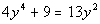Step 1:  Write in Standard Form,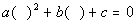, if needed.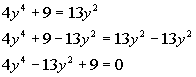*Inverse of add. 13 y squared is sub. 13 y squared  *Equation in standard form

 Below, I have the original equation rewritten in a way to show you that it is quadratic in form.  Note how when you square y squared you get y to the fourth, which is what you have in the first term.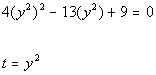*Rewriting original equation to show it is quadratic in form *Note that (y squared) squared = y to the fourth *When in stand. form, let t = the expression following b.

 Next, we need to substitute t in for y squared in the original equation.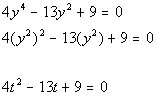*Original equation     *Substitute t in for y squared

 Note how we ended up with a quadratic equation when we did our substitution.  From here, we need to solve the quadratic equation that we have created.

 Step 3: Solve the quadratic equation created in step 2.

 You can use any method you want to solve the quadratic equation: factoring, completing the square or quadratic formula. I'm going to factor it to solve it.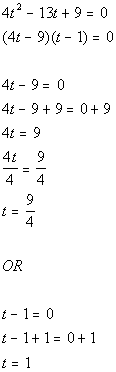*Use Zero-Product Principle *Set 1st factor = 0 and solve                   *Set 2nd factor = 0 and solve

 In step 2 we used the substitution of t = y squared.

 Let's find the value(s) of y when t = 9/4: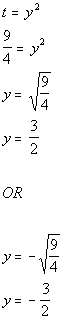*Plug in 9/4 for t *Use square root method to solve for y   *First solution               *Second solution

 Let's find the value(s) of y when t = 1: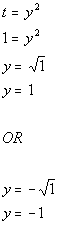*Plug in 1 for t *Use square root method to solve for y *First solution           *Second solution

 Step 5:  Check your solutions.

 Let's double check to see if y = 3/2 is a solution to the original equation.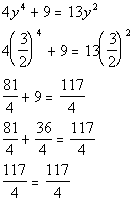*Plugging in 3/2 for y             *True statement

 Since we got a true statement, y = 3/2  is a solution.

 Let's double check to see if y = -3/2 is a solution to the original equation.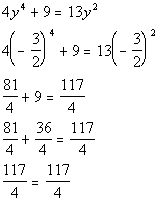*Plugging in -3/2 for y             *True statement

 Since we got a true statement, y = -3/2  is a solution.

 Let's double check to see if y = 1 is a solution to the original equation.*Plugging in 1 for y *True statement

 Since we got a true statement, y = 1  is a solution.

 Let's double check to see if y = -1 is a solution to the original equation.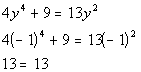*Plugging in -1 for y *True statement

 Since we got a true statement, y = -1  is a solution.

 There are four solutions to this equation: y = 3/2, y = -3/2, y = 1, and y = -1.Answer/Discussion to 1b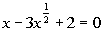Step 1:  Write in Standard Form,, if needed.

 This equation is already in standard form.

 Below, I have the original equation rewritten in a way to show you that it is quadratic in form.  Note how when you square x to the 1/2 power you get x, which is what you have in the first term.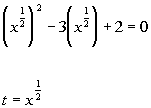*Rewriting original equation to show it is quadratic in form *Note that (x to the 1/2 power) squared = x *When in stand. form, let t = the expression following b.

 Next, we need to substitute t in for x to the 1/2 power in the original equation.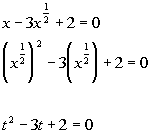*Original equation     *Substitute t in for x to the 1/2 power

 Note how we ended up with a quadratic equation when we did our substitution.  From here, we need to solve the quadratic equation that we have created.

 Step 3: Solve the quadratic equation created in step 2.

 You can use any method you want to solve the quadratic equation: factoring, completing the square or quadratic formula. I'm going to factor it to solve it.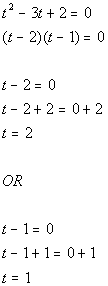*Use Zero-Product Principle *Set 1st factor = 0 and solve           *Set 2nd factor = 0 and solve

 In step 2 we used the substitution of t = x to the 1/2 power.

 Let's find the value(s) of x when t = 2: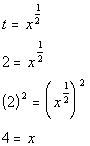*Plug in 3 for t *Solve the rational exponent equation *Inverse of taking it to the 1/3 power is raising it to the 3rd power

 Let's find the value(s) of x when t = 1:*Plug in 1 for t *Solve the rational exponent equation *Inverse of taking it to the 1/2 power is raising it to the 2nd power

 Step 5:  Check your solutions.

 Let's double check to see if x = 4 is a solution to the original equation.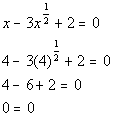*Plugging in 4 for x   *True statement

 Since we got a true statement, x = 4  is a solution.

 Let's double check to see if x = 1 is a solution to the original equation.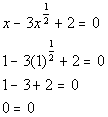*Plugging in 1 for x   *True statement

 Since we got a true statement, x = 1  is a solution.

 There are two solutions to this equation: x = 4 and x = 1.Answer/Discussion to 1c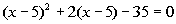Step 1:  Write in Standard Form,, if needed.

 This equation is already in standard form.

 Note how the original equation has the exact same expression in the two (  )'s and that the first (  ) is squared and the 2nd (  ) is to the one power.  This equation is quadratic in form.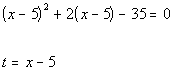*When in stand. form, let t = the expression following b.

 Next, we need to substitute t in for x minus 5 in the original equation.*Original equation   *Substitute t in for x minus 5

 Note how we ended up with a quadratic equation when we did our substitution.  From here, we need to solve the quadratic equation that we have created.

 Step 3: Solve the quadratic equation created in step 2.

 You can use any method you want to solve the quadratic equation: factoring, completing the square or quadratic formula. I'm going to factor it to solve it.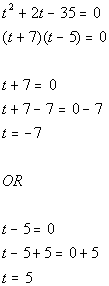*Use Zero-Product Principle *Set 1st factor = 0 and solve           *Set 2nd factor = 0 and solve

 In step 2 we used the substitution of t = x - 5.

 Let's find the value(s) of x when t = -7: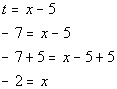*Plug in -7 for t *Inverse of sub. 5 is add. 5

 Let's find the value(s) of x when t = 5:*Plug in 5 for t *Inverse of sub. 5 is add. 5

 Step 5:  Check your solutions.

 Let's double check to see if x = -2 is a solution to the original equation.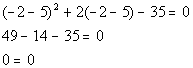*Plugging in -2 for x *True statement

 Since we got a true statement, x = -2  is a solution.

 Let's double check to see if x = 10 is a solution to the original equation.*Plugging in 10 for x *True statement

 Since we got a true statement, x = 10  is a solution.

 There are two solutions to this equation: x = -2 and x = 10.

WTAMU > Virtual Math Lab > College Algebra > Tutorial 20: Equations that are Quadratic in Form

Last revised on Dec. 16, 2009 by Kim Seward.
All contents copyright (C) 2002 - 2010, WTAMU and Kim Seward. All rights reserved.